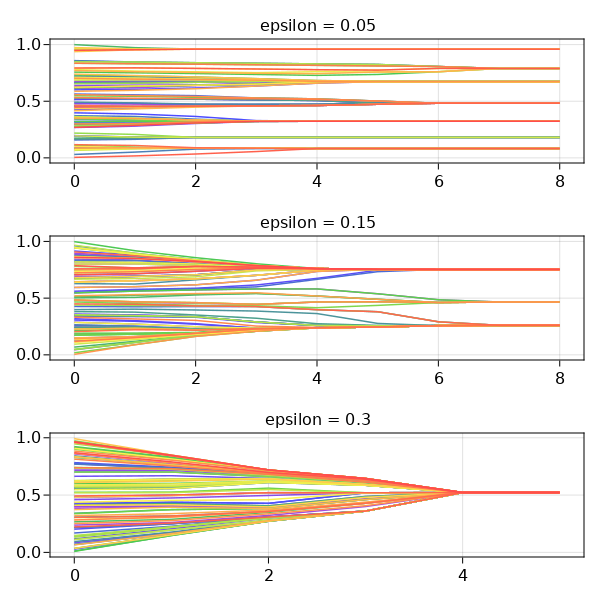HK (Hegselmann and Krause) opinion dynamics model

This example showcases

• How to do synchronous updating of Agent properties (also know as Synchronous update schedule). In a Synchronous update schedule changes made to an agent are not seen by other agents until the next step, see also Wilensky 2015, p.286).
• How to terminate the system evolution on demand according to a boolean function.
• How to terminate the system evolution according to what happened on the previous step.

Model overview

This is an implementation of a simple version of the Hegselmann and Krause (2002) model. It is a model of opinion formation with the question: which parameters' values lead to consensus, polarization or fragmentation? It models interacting groups of agents (as opposed to interacting pairs, typical in the literature) in which it is assumed that if an agent disagrees too much with the opinion of a source of influence, the source can no longer influence the agent's opinion. There is then a "bound of confidence". The model shows that the systemic configuration is heavily dependent on this parameter's value.

The model has the following components:

• A set of n Agents with opinions xᵢ in the range [0,1] as attribute
• A parameter ϵ called "bound" in (0, 0.3]
• The update rule: at each step every agent adopts the mean of the opinions which are within the confidence bound ( |xᵢ - xⱼ| ≤ ϵ).

It is also available from the Models module as Models.hk.

Core structures

We start by defining the Agent type and initializing the model. The Agent type has two fields so that we can implement the synchronous update.

using Agents
using Statistics: mean

mutable struct HKAgent <: AbstractAgent
id::Int
old_opinion::Float64
new_opinion::Float64
previous_opinion::Float64
end

There is a reason the agent has three fields that are "the same". The old_opinion is used for the synchronous agent update, since we require access to a property's value at the start of the step and the end of the step. The previous_opinion is the opinion of the agent in the previous step, as the model termination requires access to a property's value at the end of the previous step, and the end of the current step.

We could, alternatively, make the three opinions a single field with vector value.

function hk_model(; numagents = 100, ϵ = 0.2)
model = ABM(HKAgent, scheduler = Schedulers.fastest, properties = Dict(:ϵ => ϵ))
for i in 1:numagents
o = rand(model.rng)
end
return model
end

model = hk_model()
AgentBasedModel with 100 agents of type HKAgent
space: nothing (no spatial structure)
scheduler: fastest
properties: ϵ

Add some helper functions for the update rule. As there is a filter in the rule we implement it outside the agent_step! method. Notice that the filter is applied to the :old_opinion field.

function boundfilter(agent, model)
filter(
j -> abs(agent.old_opinion - j) < model.ϵ,
[a.old_opinion for a in allagents(model)],
)
end

Now we implement the agent_step!

function agent_step!(agent, model)
agent.previous_opinion = agent.old_opinion
agent.new_opinion = mean(boundfilter(agent, model))
end

and model_step!

function model_step!(model)
for a in allagents(model)
a.old_opinion = a.new_opinion
end
end

From this implementation we see that to implement synchronous scheduling we define an Agent type with old and new fields for attributes that are changed via the synchronous update. In agent_step! we use the old field then, after updating all the agents new fields, we use the model_step! to update the model for the next iteration.

Running the model

The parameter of interest is now :new_opinion, so we assign it to variable adata and pass it to the run! method to be collected in a DataFrame.

In addition, we want to run the model only until all agents have converged to an opinion. From the documentation of step! one can see that instead of specifying the amount of steps we can specify a function instead.

function terminate(model, s)
if any(
!isapprox(a.previous_opinion, a.new_opinion; rtol = 1e-12)
for a in allagents(model)
)
return false
else
return true
end
end

Agents.step!(model, agent_step!, model_step!, terminate)
model
Main.HKAgent(1, 0.6300605969631166, 0.6300605969631166, 0.6300605969631158)

Alright, let's wrap everything in a function and do some data collection using run!.

function model_run(; kwargs...)
model = hk_model(; kwargs...)
agent_data, _ = run!(model, agent_step!, model_step!, terminate; adata = [:new_opinion])
return agent_data
end

data = model_run(numagents = 100)
data[(end-19):end, :]

20 rows × 3 columns

stepidnew_opinion
Int64Int64Float64
16810.717439
26820.281391
36830.717439
46840.717439
56850.717439
66860.281391
76870.717439
86880.717439
96890.717439
106900.717439
116910.717439
126920.717439
136930.717439
146940.717439
156950.717439
166960.281391
176970.281391
186980.717439
196990.717439
2061000.281391

Notice that here we didn't speciy when to collect data, so this is done at every step. Instead, we could collect data only at the final step, by re-using the same function for the when argument:

model = hk_model()
agent_data, _ = run!(
model,
agent_step!,
model_step!,
terminate;
when = terminate,
)
agent_data

100 rows × 3 columns

stepidnew_opinion
Int64Int64Float64
1710.760685
2720.336012
3730.760685
4740.336012
5750.336012
6760.760685
7770.336012
8780.336012
9790.336012
107100.336012
117110.760685
127120.336012
137130.336012
147140.336012
157150.336012
167160.336012
177170.760685
187180.336012
197190.336012
207200.336012
217210.336012
227220.760685
237230.336012
247240.336012
257250.336012
267260.760685
277270.336012
287280.336012
297290.336012
307300.336012
317310.336012
327320.760685
337330.336012
347340.336012
357350.336012
367360.760685
377370.760685
387380.760685
397390.336012
407400.336012
417410.336012
427420.336012
437430.760685
447440.760685
457450.336012
467460.336012
477470.336012
487480.760685
497490.760685
507500.336012
517510.336012
527520.336012
537530.336012
547540.336012
557550.336012
567560.336012
577570.760685
587580.336012
597590.336012
607600.336012
617610.336012
627620.336012
637630.336012
647640.336012
657650.336012
667660.336012
677670.336012
687680.336012
697690.336012
707700.336012
717710.336012
727720.760685
737730.336012
747740.760685
757750.336012
767760.336012
777770.336012
787780.760685
797790.760685
807800.336012
817810.336012
827820.336012
837830.760685
847840.336012
857850.760685
867860.336012
877870.336012
887880.760685
897890.336012
907900.336012
917910.760685
927920.760685
937930.760685
947940.760685
957950.760685
967960.336012
977970.336012
987980.760685
997990.336012
10071000.336012

Finally we run three scenarios, collect the data and plot it.

using DataFrames, CairoMakie

plotsim(ax, data) =
for grp in groupby(data, :id)
lines!(ax, grp.step, grp.new_opinion, color = cmap[grp.id/100])
end

eps = [0.05, 0.15, 0.3]
figure = Figure(resolution = (600, 600))
for (i, e) in enumerate(eps)
ax = figure[i, 1] = Axis(figure; title = "epsilon = \$e")
e_data = model_run(ϵ = e)
plotsim(ax, e_data)
end
figure